There is a long run expression of Markov process which is known as steady-state probabilities which shows thatwhen the sample of a Markov chain cannot be reduced further. It is shown by:

Lt pij(n) = ∏j (i.e., independent of i)

Where ∏j shows the steady state and is denoted by the equation:

In the above expression we see that ∏j is the steady state because in the process of finding a probability of a state say j, in the long run the transition slowly tends to return back to ∏j .

We also have an equation as:

µijstands for expected recurrence time. This shows the mean recurrence time of each state.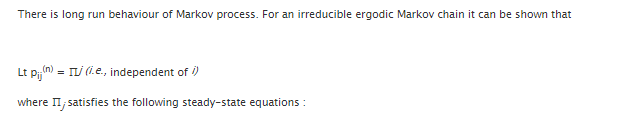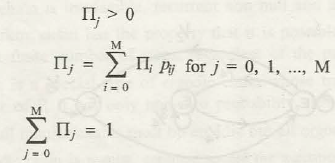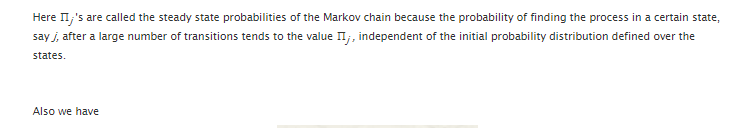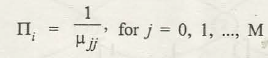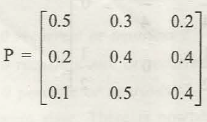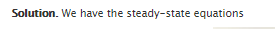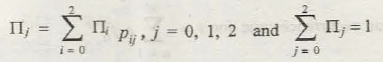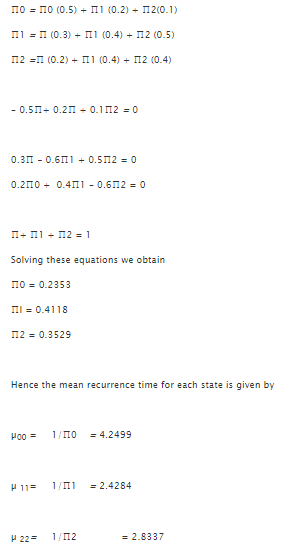### Customer Reviews

My Homework Help
Rated 5.0 out of 5 based on 510 customer reviews at